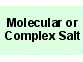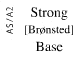Caustic potash
KOH
Potassium hydroxideH 1 K 1 O 1

Real, long lived, electronically neutral reagent chemical.

Gram formula weight (molecular mass) = 56.105
Melting point = 360 °C
Boiling point = 1327 °C
Density = 2 g/cm3
Purity = 99 %
Solubility in water = 119 g/100mL
Solubility in organics = insoluble

 Wikipedia Page:Potassium hydroxide
is defined with respect to the entities below:
The entities below are defined with respect to:
Potassium hydroxide
 Alkali (generic)Molecular ionic material (generic)Strong base (AS/A2 generic)Type 7 Lewis acid/base complex (generic)chemical compound molecule metal molecular science reaction mechanism ionic material acid base geometry reactivity synthesis science knowledge chemistry Lewis